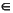MATLAB Function Referenceismember

Detect members of set

Syntax

• ```tf = ismember(A, S)
tf = ismember(A, S, 'rows')
[tf, loc] = ismember(A, S, ...)
```

Description

```tf = ismember(A, S) ``` returns a vector the same length as `A`, containing logical `1` (`true`) where the elements of `A` are in the set `S`, and logical `0` (`false`) elsewhere. In set theory terms, `k` is 1 where `A``S`. `A` and S can be cell arrays of strings.

```tf = ismember(A, S, 'rows'), ``` when `A` and `S` are matrices with the same number of columns, returns a vector containing `1` where the rows of `A` are also rows of `S` and `0` otherwise. You cannot use this syntax if `A` or `S` is a cell array of strings.

```[tf, loc] = ismember(A, S, ...) ``` returns index vector `loc` containing the highest index in `S` for each element in `A` that is a member of `S`. For those elements of `A` that do not occur in `S`, `ismember` returns `0`.

Examples

• ```set = [0 2 4 6 8 10 12 14 16 18 20];
a = reshape(1:5, [5 1])

a =
1
2
3
4
5

ismember(a, set)
ans =
0
1
0
1
0
set = [5 2 4 2 8 10 12 2 16 18 20 3];
[tf, index] = ismember(a, set);

index
index =
0
8
12
3
1
```

See Also

`issorted`, `intersect`, `setdiff`, `setxor`, `union`, `unique`, is*

© 1994-2005 The MathWorks, Inc.